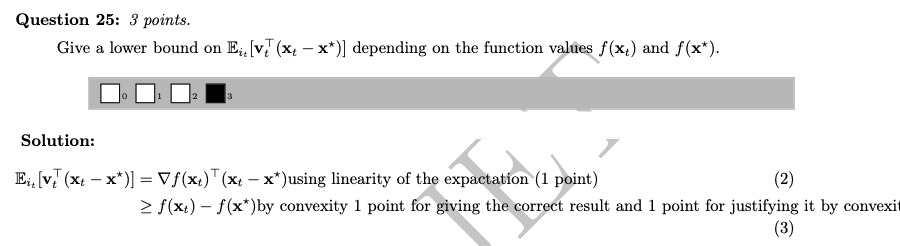### Are the succinct answers of open questions enough to answer ?

Hello,

While working on previous year's exams, I see that the solutions are very short. This is not a problem per se, but I wonder if those answers would be accepted if provided by a student. For example, during lectures, when working with stochastic gradients and expectation, we did quite a few steps to show linearity of (conditional or standard) expectation operator. Would you expect us to do that again, or can we argue just by saying that the expectaction is linear and carry on with the computations ? An example of such question would be Q24Top comment

Good question. I would recommend writing your answers are precisely and clearly as possible. While we cannot give hard criteria for what constitutes 'enough detail', this particular solution is on the short side. These solutions were written mainly to guide grading.

I see, I see, I believe we could use linearity of expectation freely at least, for example here by rewritting $$v_t$$ exactly then dealing with each term individually, then we are close to the solution and we can conclude easily.